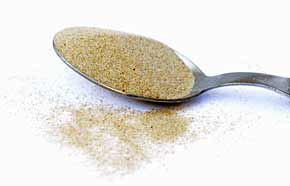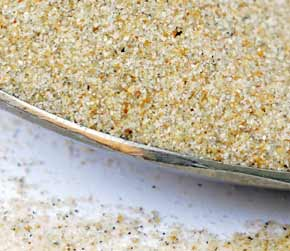﻿ Beach Sand 1 cubic inch volume to cubic decimeters converter

# beach sand conversion

## Amount: 1 cubic inch (cu in - in3) of volume Equals: 0.016 cubic decimeters (dm3) in volume

Converting cubic inch to cubic decimeters value in the beach sand units scale.

TOGGLE :   from cubic decimeters into cubic inches in the other way around.

## beach sand from cubic inch to cubic decimeter Conversion Results:

### Enter a New cubic inch Amount of beach sand to Convert From

* Whole numbers, decimals or fractions (ie: 6, 5.33, 17 3/8)
* Precision is how many numbers after decimal point (1 - 9)

Enter Amount :
Decimal Precision :

CONVERT :   between other beach sand measuring units - complete list.

Conversion calculator for webmasters.

## Beach sand weight vs. volume units

Beach sand has quite high density, it's heavy and it easily leaks into even tiny gaps or other opened spaces. No wonder it absorbs and conducts heat energy from the sun so well. However, this sand does not have the heat conductivity as high as glass does, or fireclay and firebricks, or dense concrete. A fine beach sand in dry form was used for taking these measurements.Convert beach sand measuring units between cubic inch (cu in - in3) and cubic decimeters (dm3) but in the other reverse direction from cubic decimeters into cubic inches.

 conversion result for beach sand: From Symbol Equals Result To Symbol 1 cubic inch cu in - in3 = 0.016 cubic decimeters dm3

# Converter type: beach sand measurements

This online beach sand from cu in - in3 into dm3 converter is a handy tool not just for certified or experienced professionals.

First unit: cubic inch (cu in - in3) is used for measuring volume.
Second: cubic decimeter (dm3) is unit of volume.

## beach sand per 0.016 dm3 is equivalent to 1 what?

The cubic decimeters amount 0.016 dm3 converts into 1 cu in - in3, one cubic inch. It is the EQUAL beach sand volume value of 1 cubic inch but in the cubic decimeters volume unit alternative.

How to convert 2 cubic inches (cu in - in3) of beach sand into cubic decimeters (dm3)? Is there a calculation formula?

First divide the two units variables. Then multiply the result by 2 - for example:
0.016387064 * 2 (or divide it by / 0.5)

QUESTION:
1 cu in - in3 of beach sand = ? dm3

1 cu in - in3 = 0.016 dm3 of beach sand

## Other applications for beach sand units calculator ...

With the above mentioned two-units calculating service it provides, this beach sand converter proved to be useful also as an online tool for:
1. practicing cubic inches and cubic decimeters of beach sand ( cu in - in3 vs. dm3 ) measuring values exchange.
2. beach sand amounts conversion factors - between numerous unit pairs variations.
3. working with mass density - how heavy is a volume of beach sand - values and properties.

International unit symbols for these two beach sand measurements are:

Abbreviation or prefix ( abbr. short brevis ), unit symbol, for cubic inch is:
cu in - in3
Abbreviation or prefix ( abbr. ) brevis - short unit symbol for cubic decimeter is:
dm3

### One cubic inch of beach sand converted to cubic decimeter equals to 0.016 dm3

How many cubic decimeters of beach sand are in 1 cubic inch? The answer is: The change of 1 cu in - in3 ( cubic inch ) volume unit of beach sand measure equals = to volume 0.016 dm3 ( cubic decimeter ) as the equivalent measure within the same beach sand substance type.

In principle with any measuring task, switched on professional people always ensure, and their success depends on, they get the most precise conversion results everywhere and every-time. Not only whenever possible, it's always so. Often having only a good idea ( or more ideas ) might not be perfect nor good enough solution. If there is an exact known measure in cu in - in3 - cubic inches for beach sand amount, the rule is that the cubic inch number gets converted into dm3 - cubic decimeters or any other beach sand unit absolutely exactly.

Conversion for how many cubic decimeters ( dm3 ) of beach sand are contained in a cubic inch ( 1 cu in - in3 ). Or, how much in cubic decimeters of beach sand is in 1 cubic inch? To link to this beach sand cubic inch to cubic decimeters online converter simply cut and paste the following.
The link to this tool will appear as: beach sand from cubic inch (cu in - in3) to cubic decimeters (dm3) conversion.

I've done my best to build this site for you- Please send feedback to let me know how you enjoyed visiting.